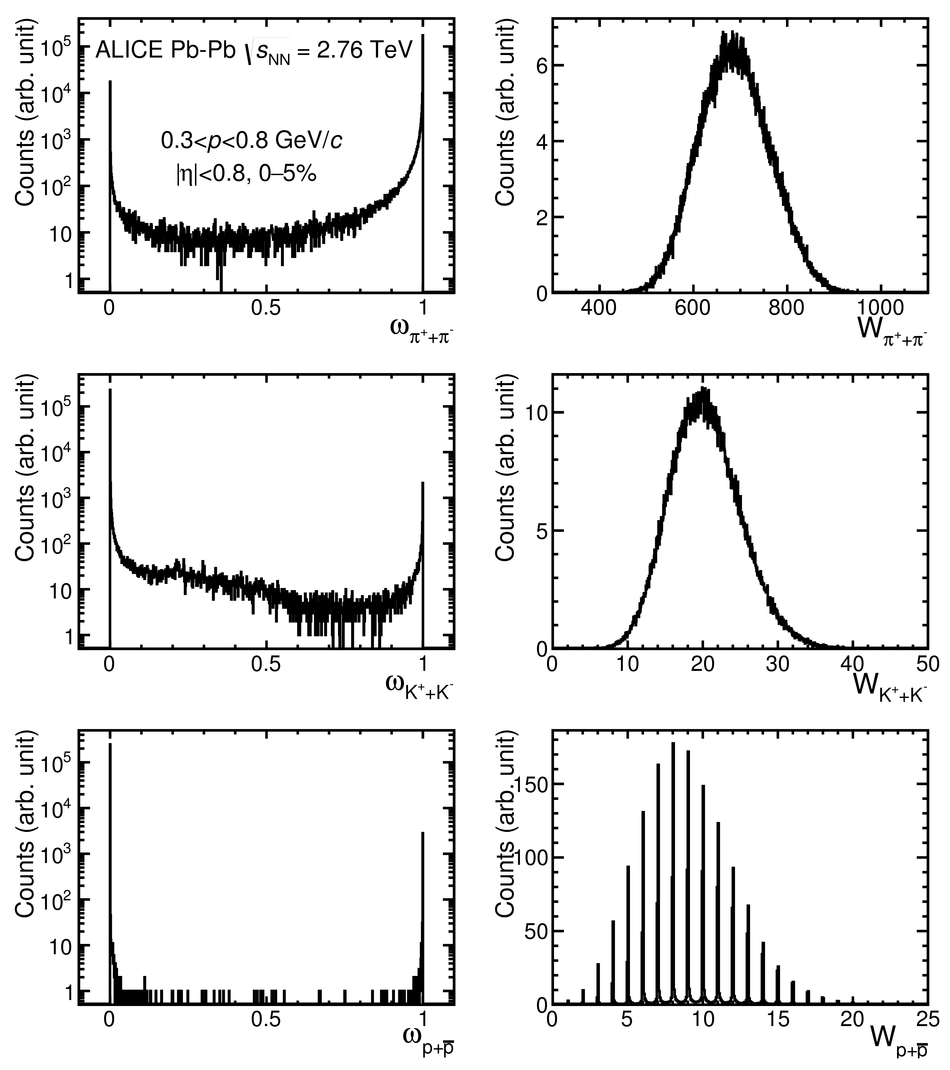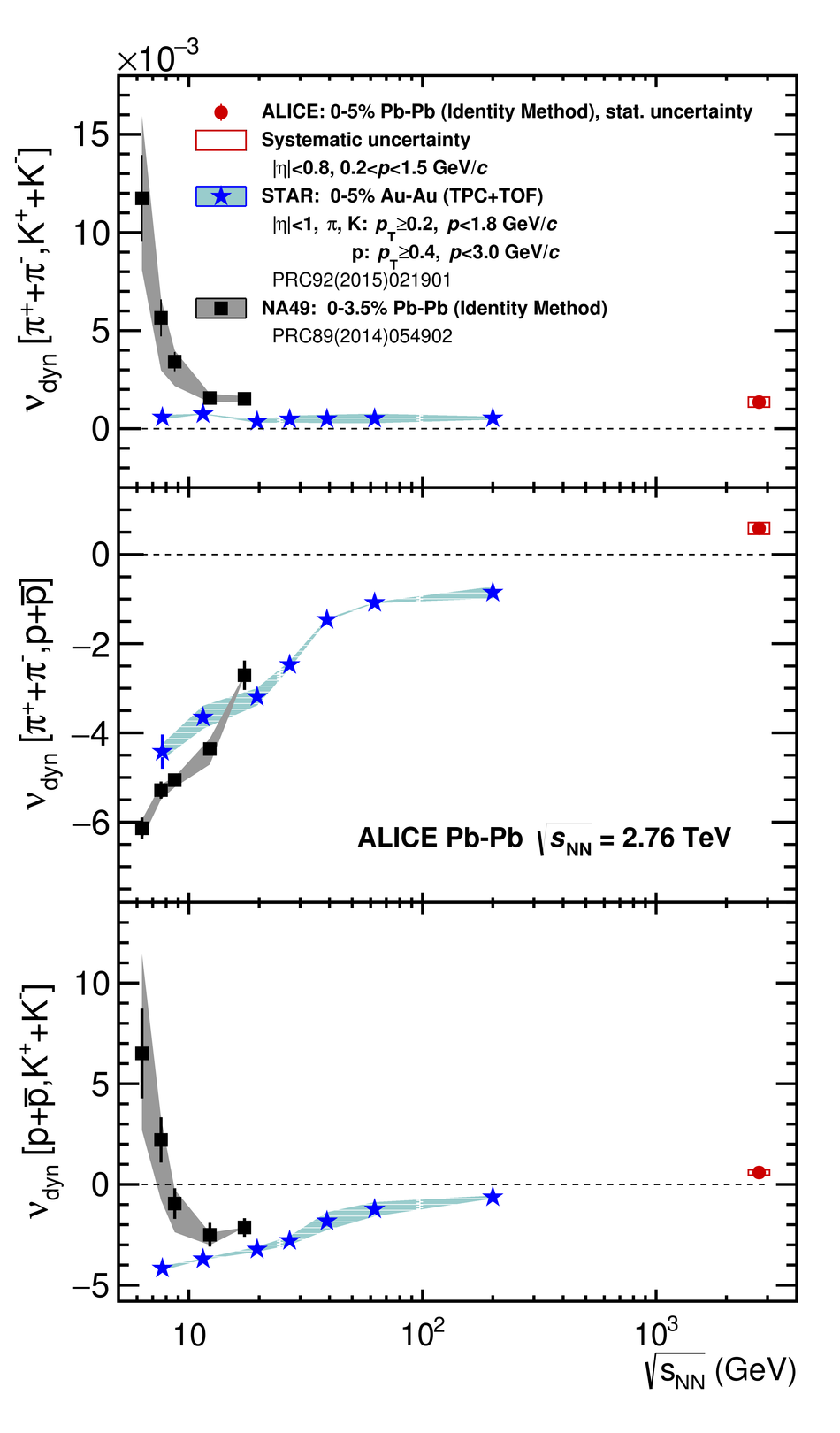# Relative particle yield fluctuations in Pb-Pb collisions at ${\mathbf{\sqrt{s_{\rm NN}}=2.76}}$ TeV

First results on K/$\pi$, p/$\pi$ and K/p fluctuations are obtained with the ALICE detector at the CERN LHC as a function of centrality in Pb-Pb collisions at $\sqrt{s_{\rm NN}}=2.76$ TeV. The observable $\nu_{\rm dyn}$, which is defined in terms of the moments of particle multiplicity distributions, is used to quantify the magnitude of dynamical fluctuations of relative particle yields and also provides insight into the correlation between particle pairs. This study is based on a novel experimental technique, called the Identity Method, which allows one to measure the moments of multiplicity distributions in case of incomplete particle identification. The results for p/$\pi$ show a change of sign in $\nu_{\rm dyn}$ from positive to negative towards more peripheral collisions. For central collisions, the results follow the smooth trend of the data at lower energies and $\nu_{\rm dyn}$ exhibits a change in sign for p/$\pi$ and K/p.

Figures

## Figure 1## Figure 2

 Distributions of the TPC \dEdx signal of pions, kaons, electrons and protons fitted with the generalized Gaussian function in a given phase-space bin. The residuals are defined as the difference between data points and the total fit function normalized to the statistical error of the data points.## Figure 3

 Results for \nudynpikashort, \nudynpiprshort and \nudynkaprshort scaled by the charged-particle density \dNdeta. The ALICE data are shown by red markers while the coloured lines indicate the HIJING and AMPT model calculations. The data are shown as a function of the collision centrality, expressed in terms of \dNdeta.## Figure 4

 Collision-energy dependence of \nudyn. Results obtained with the Identity Method in this work and by the NA49 collaboration in Pb--Pb collisions are shown with red circles and black squares, respectively, while those obtained by the STAR collaboration in Au--Au collisions are shown with blue stars.